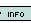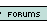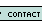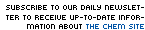1. There are two beakers sitting on a table. One contains 56.20 grams of water. The other contains hydrochloric acid (HCl). The number of atoms of hydrogen in the HCl equals the number of total atoms in the beaker full of water. How many grams of HCl are in the second beaker? 341.4 grams 142.4 g 938.1 g 53.29 g None of these 2. When aluminum reacts with sulfuric acid, the result is aluminum sulfate and hydrogen gas. How many moles of sulfuric acid (H2SO4) will react with 24 moles of aluminum? 12 14 16 18 20 3. How many carbon molecules are present in 5.0 grams of glucose? (C6H12O6) 1.0 * 10^22 5.1 * 10^22 1.0 * 10^23 6 None 4. How many kilometers are in 10.00 miles? (1 inch equals exactly 2.54 centimeters) 2.04 km 4.09 km 8.09 km 16.09 km None of these 5. Substance A combines with substance B to form Substance AB. B is a diatomic molecule. You are given 3.15 moles of B2. If A is in excess, how many moles of AB are produced? 1.20 2.30 3.25 5.60 6.30 6. There are 2.34 moles of substance A3 combined with 2.77 moles of substance B. Assume that the reaction goes to completion. After the reaction is complete, AB is reacted with substance C2. The reaction creates CB and A. If C2 was in excess during the second reaction, then how many moles of CB were produced? 2.43 moles 3.82 moles 4.38 moles 7.02 moles None of these 7. How many moles in 20 g of water? 1.11 20 18 1.1 42 8. How many grams is 0.25 moles of table salt (NaCl?) 58.44 grams 14.61 grams 14.6 grams 58 grams 15 grams 9. The empirical formula of a compound is C10H20 with a molar mass of 284 grams, find the molecular weight. C20H40 C10H20 C5H10 C4H8 CH4 10. On a faraway planet, the following system of measurement is used: 1 zorg = 5.81 zaks 1 zak = .87 zips 1 zip = 2.52 zeeks 1 zeek = 10.02 yaks 1 yak = 3.91 zaps How many zorgs are in 3.20 zaps? 5.81 12.53 2.74 0.00641 None of these 11. A chemistry student was performing an experiment involving the following reaction: C3H8 (g) + O2 (g) --> CO2 (g) + H2O (g), balancing it with the coefficients 1, 5, 2, and 4 respectively. The oxygen was in excess. He used 20.18 grams of C3H8. When he measured the amount of CO2 produced, he was astounded to find that 46.22 grams were produced, 5.88 grams more than the theoretical yield of 40.34. What went wrong? Hint: Look at the formula C3H8's coefficient should be 3, not 1 CO2's coefficient should be 3, not 2 O2's coefficient should be 3, not 5 Water's coefficient should be 3, not 4 None of these 12. 6.02x1023 is.... a random number Avogadro's number stoichiometry percent yield None of these 13. What is the mass percent of oxygen in H2SO4 (sulfuric acid)? 0 1% 2.04% 32.70% 65.26% 14. When 2 moles of propane (C3H8, MW = 44 grams) is combusted with oxygen gas, how many grams of CO2 (MW: 44 grams) are produced? 88 44 3 132 264 15. The formation of ammonia is shown by the following reaction: N2 (g) + 3H2 (g)--> 2NH3 (g). You are given 13.85 grams of nitrogen and 5.27 grams of hydrogen. If the experiment produced 3.297 grams of ammonia, then what is the percent yield? 25% 50% 35.80% 76.60% None of these Name: E-mail: Last 15 Challengersnews | about us | contact us tutorials index | organic chemistry | practice tests | online quizzes | reference tools site copyright (c) 2002 to neopages.com